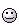A Flash Developer Resource Site

# Thread: throwing & dragging objects...

1. ## throwing & dragging objects...

Hi,
I am trying to complete a drag and drop feature with multiple objects. I can get a single object to work perfectly... it drags and opens to another screen when double clicked. Trouble is when I try to add another object only one will work.... Please help, this is causing me major headaches!!!
Here's the code I've got....

dragging = false;
friction = 0.98;
bounce = -0.7;
gravity = 0.5;
top = 0;
left = 0;
bottom = Stage.height;
right = Stage.width;

pic._x = Stage.width / 2;
pic._y = Stage.height / 2;
vx = Math.random() * 10 - 5;
vy = Math.random() * 10 - 5;

pic.onPress = function()
{
oldX = pic._x;
oldY = pic._y;
dragging = true;
pic.startDrag();
};
pic.onRelease = function()
{
dragging = false;
pic.stopDrag();
if (C1) {
if(getTimer() < t1+500){
C1 = 0;
getURL ("http://www.eyekiller.com/flash.htm")
}
} else {
C1 = 1;
t1 = getTimer();
}
};
pic.onReleaseOutside = function()
{
dragging = false;
pic.stopDrag();
};
this.onEnterFrame=function()
{
if(C1 && getTimer() >= t1+500){
C1 = 0;
}
if (!dragging)
{

vx *= friction;
vy *= friction;
pic._x += vx;
pic._y += vy;
if (pic._x + pic._width / 2 > right)
{
pic._x = right - pic._width / 2;
vx *= bounce;
}
else if (pic._x - pic._width / 2 < left)
{
pic._x = left + picl._width / 2;
vx *= bounce;
}
if (pic._y + pic._height / 2 > bottom)
{
pic._y = bottom - pic._height / 2;
vy *= bounce;
}
else if (pic._y - pic._height / 2 < top)
{
pic._y = top + pic._height / 2;
vy *= bounce;
}
}
else
{
vx = pic._x - oldX;
vy = pic._y - oldY;
oldX = pic._x;
oldY = pic._y;
}
};

dragging = false;
friction = 0.98;
bounce = -0.7;
gravity = 0.5;
top = 0;
left = 0;
bottom = Stage.height;
right = Stage.width;

ball._x = Stage.width / 2;
ball._y = Stage.height / 2;
vx = Math.random() * 10 - 5;
vy = Math.random() * 10 - 5;

ball.onPress = function()
{
oldX = ball._x;
oldY = ball._y;
dragging = true;
ball.startDrag();
};
ball.onRelease = function()
{
dragging = false;
ball.stopDrag();
if (C1) {
if(getTimer() < t1+500){
C1 = 0;
getURL ("http://www.eyekiller.com/flash.htm")
}
} else {
C1 = 1;
t1 = getTimer();
}
};
ball.onReleaseOutside = function()
{
dragging = false;
ball.stopDrag();
};
this.onEnterFrame=function()
{
if(C1 && getTimer() >= t1+500){
C1 = 0;
}
if (!dragging)
{

vx *= friction;
vy *= friction;
ball._x += vx;
ball._y += vy;
if (ball._x + ball._width / 2 > right)
{
ball._x = right - ball._width / 2;
vx *= bounce;
}
else if (ball._x - ball._width / 2 < left)
{
ball._x = left + ball._width / 2;
vx *= bounce;
}
if (ball._y + ball._height / 2 > bottom)
{
ball._y = bottom - ball._height / 2;
vy *= bounce;
}
else if (ball._y - ball._height / 2 < top)
{
ball._y = top + ball._height / 2;
vy *= bounce;
}
}
else
{
vx = ball._x - oldX;
vy = ball._y - oldY;
oldX = ball._x;
oldY = ball._y;
}
};Reply With Quote

2. havent gone through your code..but have a look at my dragging and throwing balls at http://experiments.flashmatics.co.uk

and let me know if you lookign for something like thatReply With Quote

3. Yes the boucing balls are similar. With mine I have added a little fiction and gravity so the user can 'throw' the balls around. I also require the user to be able to double click on each object so it will open another page etc. This all works fine and if I can solve the problem of multiple objects i'am almost there!
You have many bouncing objects and if I could add the extra features I need it could very well do the trick!!

What do I do next?Reply With Quote

4. the bouncing balls are throwable as well. What i'll do is send u the fla when i get home and if u have any questions regarding it.. u can gimme a shout backReply With Quote

5. Fantastic thats great!
Hear from you soon, thanks!Reply With Quote

6. ## boucing balls fla...

Hi sorry to hassle you, but could you send me the bouncing balls fla so i can have a peak & see if it will do the trick.

Many many thanks

GReply With Quote

7. here u go mate.. hope it helpsReply With Quote

8. Thanks, I'll give it a go & let you know how I get on...
Cheers!!Reply With Quote

####Posting Permissions

• You may not post new threads
• You may not post replies
• You may not post attachments
• You may not edit your posts
•

 » Home » Movies » Tutorials » Submissions » Board » Links » Reviews » Feedback » Gallery » Fonts » The Lounge » Sound Loops » Sound FX » About FK » Sitemap